# 二叉排序树(BST)

## 定义

1. 若左子树不空，则左子树上所有结点的值均小于它的根结点的值；
2. 若右子树不空，则右子树上所有结点的值均大于它的根结点的值；
3. 左、右子树也分别为二叉排序树；

## 代码实现-基本结构搭建

### 节点类编写

class Node {
int value;
Node left;
Node right;
public Node(int value) {
this.value = value;
}
// 中序遍历
public void infixOrder() {
if (this.left != null) {
this.left.infixOrder();
}
System.out.println(this);
if (this.right != null) {
this.right.infixOrder();
}
}
@Override
public String toString() {
return "Node{" +
"value=" + value +
'}';
}
}


### 二叉排序树类编写

class BinarySortTree {
private Node root;
// 中序遍历
public void infixOrder() {
if (root != null) {
root.infixOrder();
} else {
System.out.println("树为空！");
}
}
}


## 代码实现-添加方法

### 思想

1. 如果待添加节点比当前节点小
2. 判断当前节点是否为空，为空直接将待添加节点添加到当前节点左边
3. 如果不为空则将左子节点当作当前节点继续进行第一步
4. 如果待添加节点比当前节点大
5. 判断当前节点是否为空，为空直接将待添加节点添加到当前节点右
6. 如果不为空则将右子节点当作当前节点继续进行第一步

### 图示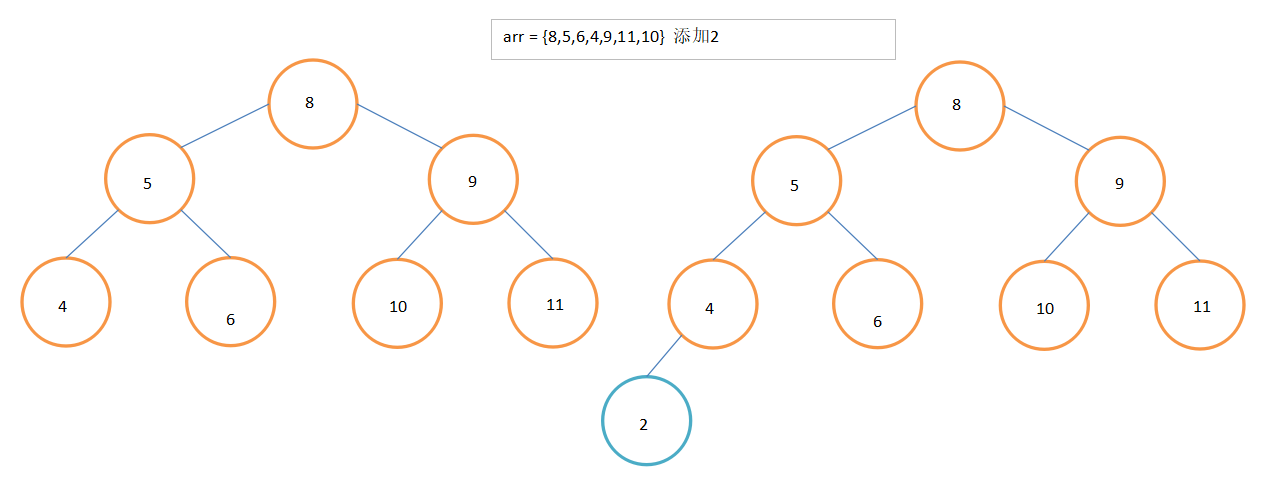### 节点类代码

public void add(Node node) {
if (node == null) {
return;
}
// 传入节点的值，和当前子树的根节点的关系
if (node.value < this.value) {
if (this.left == null) {
this.left = node;
} else {
}
} else {
if (this.right == null) {
this.right = node;
} else {
}
}
}


### 二叉排序树类代码

public void add(Node node) {
if (root == null) {
root = node;
} else {
}
}


## 代码实现-删除代码

### 思想

1. 查找到target和parent
2. 判断target是parent的左子树还是右子树
3. 若为左子树则:parent.left=null
4. 若为右子树则:parent.right=null

1. 查找到target和parent
2. 若target有左子树target.left
1. 如果target是parent的左子树则:parent.left = target.left
2. 如果target是parent的右子树则:parent.right = target.left
3. 若target有右子树target.right
1. 如果target是parent的左子树则:parent.left = target.rigth
2. 如果target是parent的右子树则:parent.right = target.right

1. 查找到target
2. 存储target的左子树的最大节点，或者存储target的右子树的最小(下面的代码中先会以右子树的最小节点为例，但是后面的课后练习中会再补上左子树最大的代码)
3. 删除掉存储的节点
4. 将target替换为存储的节点target.value = temp

### 辅助方法编写

#### 1. 查找target节点

public Node search(int value) {
if (value == this.value) {
return this;
} else if(value < this.value) {
// 查找的值小于当前节点，向左子树递归查找
// 如果左子节点为空,就代表找不到了
if (this.left == null) {
return null;
}
return this.left.search(value);
} else {
// 查找的值不小于
if (this.right == null) {
return null;
}
return this.right.search(value);
}
}


// 查找要删除的节点
public Node search(int value) {
if (root == null) {
return null;
} else {
return root.search(value);
}
}


#### 2. 查找parent节点

/**
* 查找要删除节点的父节点
* @param value 要查找的值
* @return 返回是查找的值的父节点
*/
public Node searchParent(int value) {
if ((this.left != null && this.left.value == value) ||
(this.right != null && this.right.value == value)) {
return this;
} else {
// 如果查找的值小于当前节点的值，且当前节点的左子节点不为空
if (value < this.value && this.left!= null) {
return this.left.searchParent(value);
} else if (value >= this.value && this.right != null) {
return this.right.searchParent(value);
} else {
return null;
}
}
}


// 查找要删除的节点的父节点
public Node searchParent(int value) {
if (root == null) {
return null;
} else {
return root.searchParent(value);
}
}


#### 3. 查找传入节点的最小节点返回并删除

/**
* 删除并返回传入节点的最子小节点的值
* @param node 传入的节点
* @return 传入节点最小子节点值
*/
public int delRightTreeMin(Node node) {
Node target = node;
// 循环的查找左节点就会找到最小值
while (target.left != null) {
target = target.left;
}
// 这时候target就指向了最小的节点
// 删除最小节点
delNode(target.value);
return target.value;
}


### 删除方法代码

public void delNode(int value) {
if (root == null) {
return;
}
// 找到要删除的节点
Node targetNode = search(value);
// 如果没有找到
if (targetNode == null) {
return;
}
// 树中只有一个节点
if (root.left == null && root.right == null) {
root = null;
return;
}
Node parent = searchParent(value);
// 如果要删除的节点是叶子节点
if (targetNode.left == null && targetNode.right == null) {
if (parent.left != null && targetNode == parent.left) {
parent.left = null;
}
if (parent.right != null && targetNode == parent.right) {
parent.right = null;
}
} else if (targetNode.left != null && targetNode.right != null) {
// 有两颗子树
targetNode.value = delRightTreeMin(targetNode.right);
} else {
// 只有一个子树
// 如果要删除的节点有左子节点
if (targetNode.left != null) {
if (parent == null) {
root = targetNode.left;
return;
}
if (parent.left != null && targetNode == parent.left) {
parent.left = targetNode.left;
}
if (parent.right != null && targetNode == parent.right) {
parent.right = targetNode.left;
}
} else {
if (parent == null) {
root = targetNode.right;
return;
}
if (parent.left != null && targetNode == parent.left) {
parent.left = targetNode.right;
}
if (parent.right != null && targetNode == parent.right) {
parent.right = targetNode.right;
}
}
}
}


### 课后作业-实现删除两个子树的节点的第二种方案

/**
* 删除并返回传入节点的最大子节点的值
* @param node 传入的节点
* @return 传入节点最大子节点值
*/
public int delLeftTreeMax(Node node) {
Node target = node;
// 循环的查找左节点就会找到最小值
while (target.right != null) {
target = target.right;
}
// 这时候target就指向了最小的节点
// 删除最小节点
delNode(target.value);
return target.value;
}


targetNode.value = delLeftTreeMax(targetNode.left);


# 二叉平衡排序树(AVL)

## 引出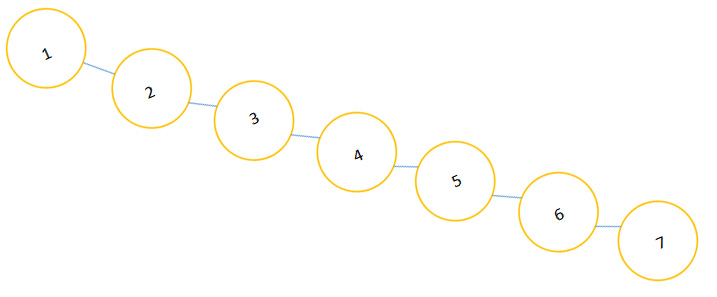## 思路

### 左旋

1. 新建一个节点为newNode，存储当前节点
2. 将newNode的左子树设为当前节点的左子树
3. 将newNode的右子树设为当前节点的右子树的左子树
4. 将当前节点的值替换为当前节点的右子树的值
5. 将当前节点的左子树设置为newNode
6. 将当前节点的右子树设置为当前节点的右子树的右子树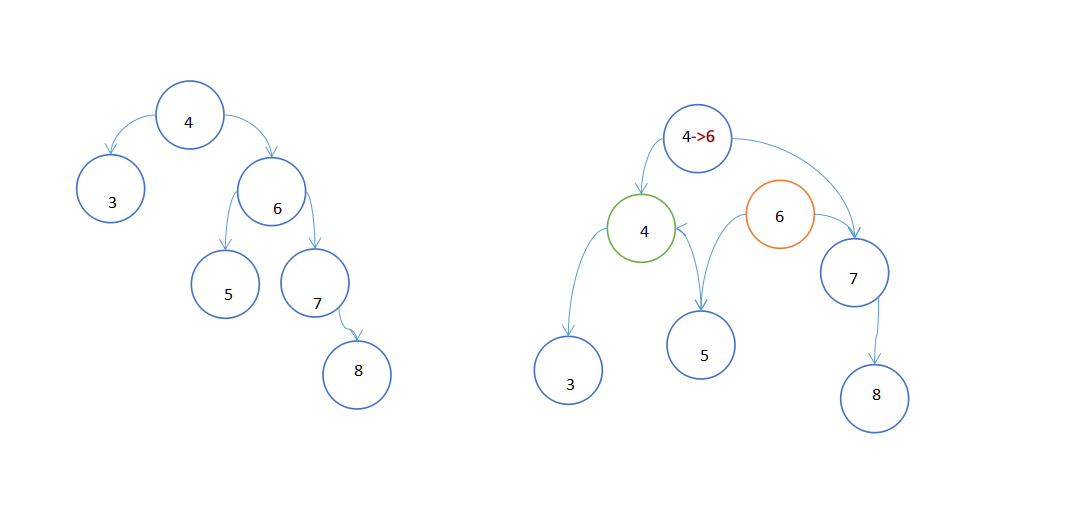图中右树的绿色框内是新建的节点，橘色框中是当前节点的右子节点。

### 右旋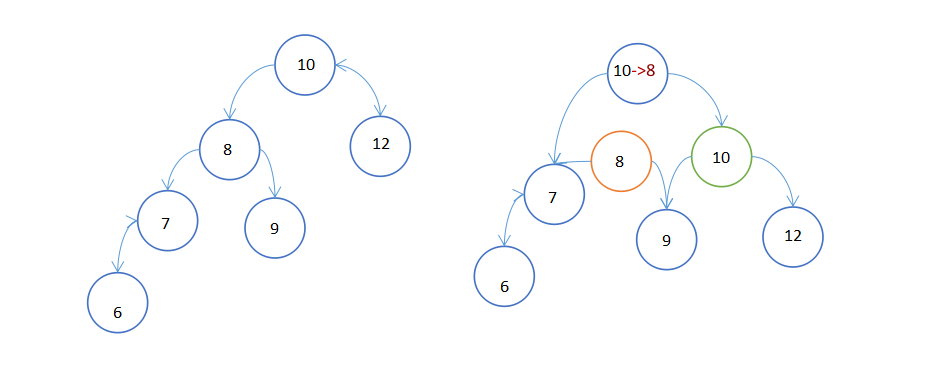### 双旋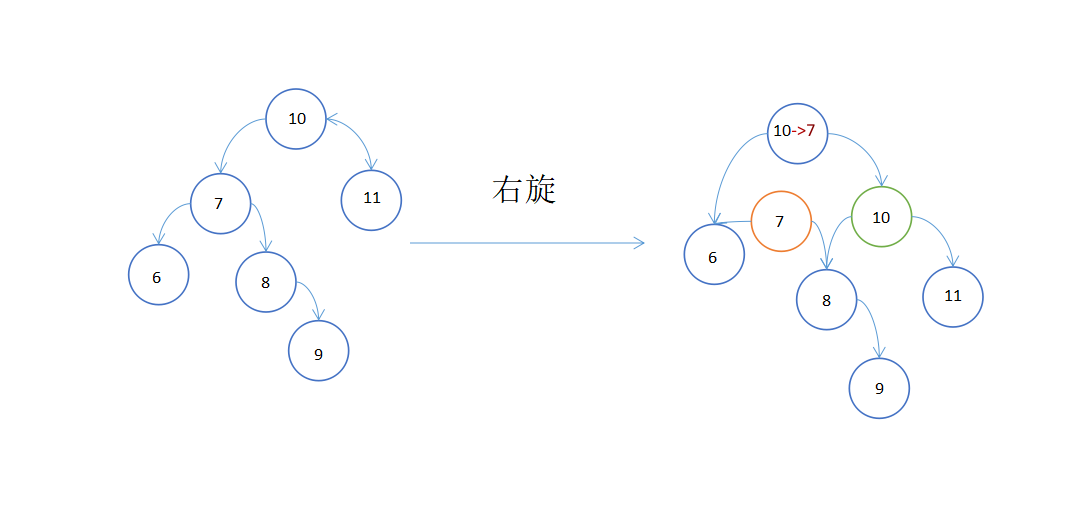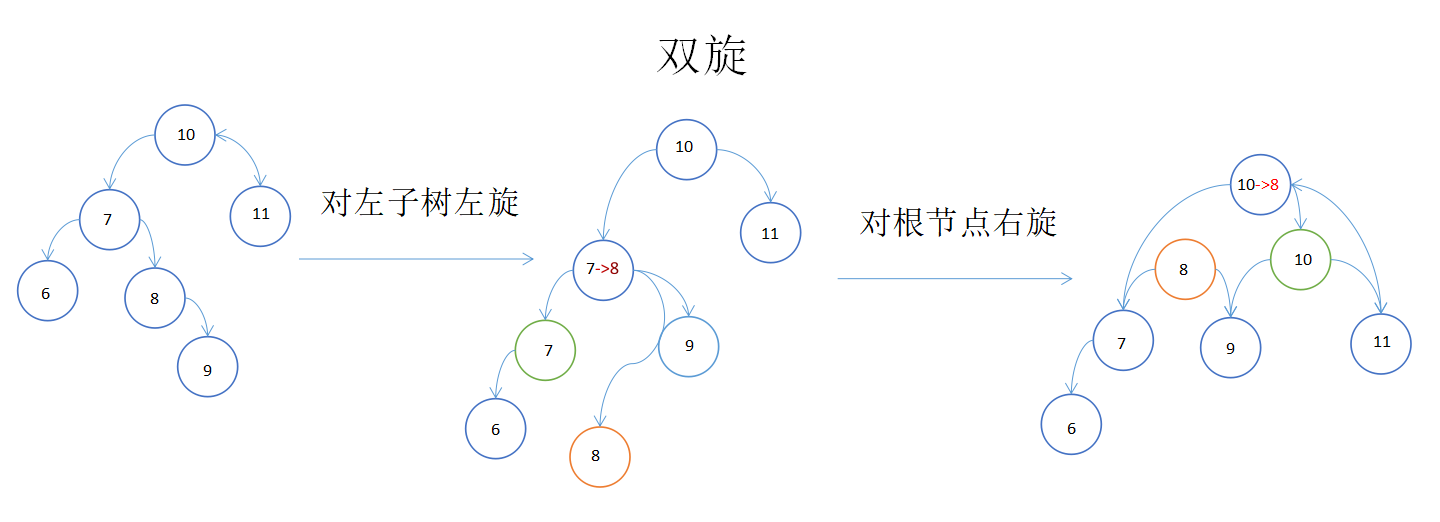## 代码实现

### 求树的高度

// 返回当前节点的高度,该节点为根节点的高度
public int height() {
return Math.max(left == null ? 0 : left.height(), right == null ? 0 : right.height()) + 1;
}

// 返回左子树的高度
public int leftHeight() {
if (left == null) {
return 0;
}
return left.height();
}

// 返回右子树的高度
public int rightHeight() {
if (right == null) {
return 0;
}
return right.height();
}


### 左旋右旋实现

// 左旋
private void leftRotate() {
// 创建新的节点，以当前根节点的值
Node newNode = new Node(value);
// 把新的节点的左子树，设置成当前节点的左子树
newNode.left = left;
// 把新的节点的右子树设置成当前节点的右子树的左子树
newNode.right = right.left;
// 把当前节点的值替换成右子节点的值
value = right.value;
// 把当前节点的右子树设置成右子树的右子树
right = right.right;
// 把当前节点的左子节点设置为新的节点
left = newNode;
}
public void rightRotate() {
Node newNode = new Node(value);
newNode.right = right;
newNode.left = left.right;
value = left.value;
left = left.left;
right = newNode;
}


public void add(Node node) {
if (node == null) {
return;
}
// 传入节点的值，和当前子树的根节点的关系
if (node.value < this.value) {
if (this.left == null) {
this.left = node;
} else {
}
} else {
if (this.right == null) {
this.right = node;
} else {
}
}
// 这之后的代码时保持平衡的代码
// 当添加完一个节点后，如果右子树的高度-左子树的高度>1 左旋转
if (rightHeight() - leftHeight() > 1) {
if (right != null && right.leftHeight() > right.rightHeight()) {
right.rightRotate(); // 右旋转
}
leftRotate(); // 左旋转
return;
}
if (leftHeight() - rightHeight() > 1) {
if (left != null && left.rightHeight() > left.leftHeight()) {
left.leftRotate(); // 左旋转
}
rightRotate(); // 右旋转
}
}
`

Q.E.D.